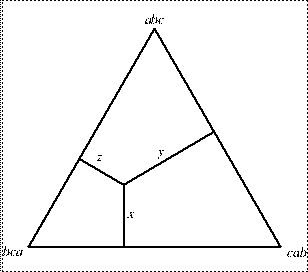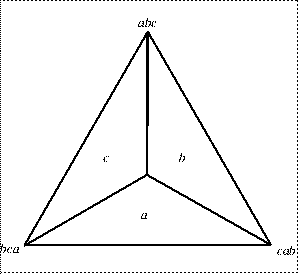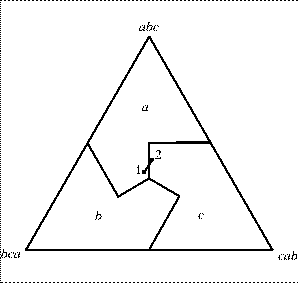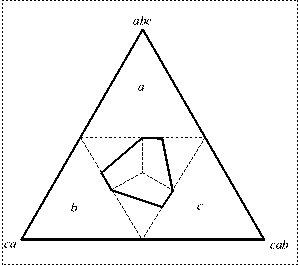Up: Issue 4 Previous: Paper 4

# Voting matters - Issue 4, August 1995

## Monotonicity - An In-Depth Study of One Example

#### D R Woodall

Douglas Woodall is Reader in Pure Mathematics at Nottingham University.

Here is a fairly typical example of the way in which monotonicity can fail with STV (or, as in this case, AV). Consider the pair of single-seat elections below. In Election 1, no candidate has reached the quota of 15, and so c, the candidate with the smallest number of first-preference votes, is excluded. All c's votes are transferred to a, and so a is elected. However, just before the result is announced, it is discovered that two of the ballots placed in the pile labelled bca are not in fact marked bca at all, but abc, so that the true situation is as in Election 2. Naturally a is delighted with this increased support. But now b has the smallest number of first-preference votes, and so, when the count is redone, b is excluded instead of c. All b's votes go to c, and so c is elected instead of a. So the effect of this increased support for a is to cause a not to be elected.

```        Election 1   Election 2
abc         11          13
bca         10           8
cab          9           9
Excluded     c           b
Elected      a           c
```
This is the sort of anomaly that has caused some people to reject the whole idea of STV. The question I want to discuss here is, how serious is it really? Certainly nobody is going to pretend that it is desirable; but is it really as bad as some people have been making out?

The first thing to notice is that nobody has been wrongly elected. One might object that it cannot possibly be the case that a is the right person to elect in Election 1 and that c is the right person to elect in Election 2, in which a clearly has more support. But it does not really make sense to talk about "the right person to elect" in these elections. In Election 1, for example, there are 19 voters who prefer c to a, and only 11 who prefer a to c, so that c seems a better candidate to elect than a. But then there are 21 voters who prefer b to c, and only 9 who prefer c to b, and so b seems a better candidate to elect than c. But then again, there are 20 voters who prefer a to b, and only 10 who prefer b to a, and so a seems a better candidate to elect than b. Whichever candidate you choose to elect, someone else can claim to be better! (Of course, this is just an example of the famous Condorcet paradox.) In this situation one should not talk about which is the right candidate to elect, but, rather, about which candidates it would be permissible to elect. It seems to me that in either of these elections it would be perfectly permissible to elect any one of the three candidates. In this situation STV really does no more than make a somewhat arbitrary selection from among the permissible candidates. It is certainly unfortunate that it chooses a in Election 1 and c in Election 2, where a clearly has more support; but it is in the nature of such processes that this sort of thing will happen.Figure 1

Let us examine more closely what is going on here. Because there are only three different types of ballot present, we can represent the situation diagrammatically, using what are known as barycentric coordinates in a triangle. Suppose we draw an equilateral triangle of unit height (Figure 1). If we put a point inside the triangle and drop perpendiculars from it, of lengths x, y and z, to the three sides of the triangle, then it is easy to prove that x + y + z = 1, the height of the triangle. So if we label the three corners of the triangle with the three different types of ballot, as in Figure 1, then we can use the point depicted to represent an election in which the proportion of voters voting abc is x, the proportion voting bca is y, and the proportion voting cab is z. Thus, for example, the top vertex of the triangle represents an election in which all the voters vote abc; the mid-point of the left side represents an election in which half vote abc and half vote bca; and so on.Figure 2: candidate excluded

Suppose now that we exclude the candidate with the smallest number of first-preference votes. Figure 2 shows which candidate is excluded. For example, to the right of the vertical line through abc there are more cab than bca ballots; and above the middle of the three lines through cab there are more abc than bca ballots. So in the region marked b, there are fewer bca ballots than ballots of either of the other two types, which means that b has fewest first-preference votes. Similar remarks apply to the other two regions.Figure 3: candidate elected

Now consider what happens if b is excluded. All of b's votes are transferred to c. So the only way that a can win is if more than half the ballots are marked abc; that is, we are above a horizontal line drawn half way up the triangle. (Of course, in this case a will be elected outright - one would not normally exclude b first; but it would make no difference to the outcome if one did.) Similar remarks apply to the other two regions, and so the result of the election is as indicated in Figure 3. Figure 3 also shows the points representing Elections 1 and 2. Election 1 is in the region where a is elected. Election 2 is obtained from it by converting two bca ballots into abc, hence by moving parallel to the left edge of the triangle. This takes us into the region in which c is elected. Of course, if one continues a bit further in the same direction, then one gets back into the region in which a is elected.

The problem is caused, in a sense, by the fact that the regions are not convex. However, one cannot make them convex without violating the spirit of STV. Their convexity is equivalent to the property called Convexity in Woodall; and, as mentioned there, the only election rules that possess this property are point-scoring systems, which do not conform to the spirit of STV.Figure 4: where monotonicity fails

This representation also gives us a way of visualizing where monotonicity fails. If there are two elections (involving only these three types of ballot) that between them show this type of failure of monotonicity, then both elections must lie inside the central region indicated in Figure 4. Note that this region is completely contained within the large dotted triangle, which is where the Condorcet paradox arises. So, in this example, monotonicity does not fail except when there is a Condorcet paradox. However, it is important to stress that, in general, monotonicity can fail even when there is no Condorcet paradox.

Figure 4 suggests the following interpretation. There are certain regions in which it is quite clear who ought to be elected, and in these regions STV elects the candidate that one would expect. But in the middle there is a grey area, where it is not at all clear who ought to be elected, and it is in this grey area that STV behaves in a somewhat haphazard manner; it is really doing no more than making a pseudo-random selection from the appropriate candidates, and it is here that small changes in the profile of ballots can cause perverse changes in the result.

The effect of this is to blur the result of an STV election. Nobody is being wrongly elected, because the problem only arises in the region where one cannot say for certain who ought to be elected anyway. And there is no systematic bias that would, for example, favour one political party rather than another. But the accuracy with which the person or persons elected in an STV election can be said to represent the views of the voters is less precise than it would be if this sort of anomaly did not arise.

The obvious question at this point is whether one can find a system that retains the essential features of STV while avoiding this sort of anomaly. The answer depends on what one regards as the essential features of STV. As we shall see in a later article, it is not possible to avoid this anomaly without sacrificing at least one property that many supporters of STV regard as essential. Nevertheless, I shall describe there a system for single-seat elections that gains so many forms of monotonicity, while sacrificing only one property of STV, that I personally would be willing to recommend it as a better system than the Alternative Vote. Unfortunately, it is not feasible when the votes are to be counted by hand. Also, it is not clear whether it can be extended in any sensible way to multi-seat elections; this is a crucial question, which I have so far been unable to answer.

### Reference

1. D R Woodall, Properties of preferential election rules, Voting matters Issue 3 (1994), 8-15.

Up: Issue 4 Previous: Paper 4